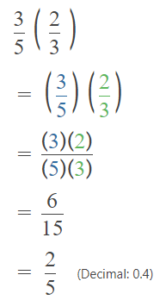# Math in Focus Grade 5 Chapter 4 Practice 2 Answer Key Real-World Problems: Multiplying with Proper Fractions

Go through the Math in Focus Grade 5 Workbook Answer Key Chapter 4 Practice 2 Real-World Problems: Multiplying with Proper Fractions to finish your assignments.

## Math in Focus Grade 5 Chapter 4 Practice 2 Answer Key Real-World Problems: Multiplying with Proper Fractions

Question 1.
Lena has some eggs in the refrigerator. She takes out $$\frac{3}{5}$$ of the eggs to make waffles and scrambled eggs. She uses $$\frac{2}{3}$$ of the eggs she took out to make waffles. What fraction of the total number of eggs does Lena use to make waffles?
$$\frac{2}{5}$$
Explanation:
Lena has some eggs in the refrigerator.
She takes out $$\frac{3}{5}$$ of the eggs to make waffles and scrambled eggs.
She uses $$\frac{2}{3}$$ of the eggs she took out to make waffles.
$$\frac{3}{5}$$ x $$\frac{2}{3}$$$$\frac{2}{5}$$ of the total number of eggs that Lena use to make waffles

Question 2.
Dawn has $$\frac{5}{6}$$ yard of lace. She uses $$\frac{4}{5}$$ of it for a dress and the rest for a jewel box. How much lace does she use for the jewel box?
$$\frac{1}{6}$$ Lace use for the jewel box
Explanation:
$$\frac{4}{5}$$ x $$\frac{5}{6}$$ = $$\frac{20}{30}$$ = $$\frac{2}{3}$$
$$\frac{5}{6}$$ – $$\frac{2}{3}$$ = $$\frac{5-4}{6}$$ = $$\frac{1}{6}$$

Question 3.
Tasha finished a job in $$\frac{3}{4}$$ hour. Megan finished it in of the time Tasha took. How long did Megan take to finish the job?
45 minutes
Explanation:
Tasha finished a job in $$\frac{3}{4}$$ hour.
Megan finished it in of the time Tasha took.
$$\frac{3}{4}$$ x 60 = 45 minutes
45 minutes Megan take to finish the job

Question 4.
Lily has a bottle containing $$\frac{7}{8}$$ quart of milk. She pours $$\frac{4}{5}$$ of it into a bowl. What amount of milk does she pour into the bowl?
$$\frac{7}{10}$$
Explanation:
Lily has a bottle containing $$\frac{7}{8}$$ quart of milk.
She pours $$\frac{4}{5}$$ of it into a bowl.
$$\frac{7}{8}$$ x $$\frac{4}{5}$$
= $$\frac{28}{40}$$
= $$\frac{7}{10}$$
$$\frac{7}{10}$$ amount of milk that she pour into the bowl

Question 5.
Raoul ran $$\frac{3}{4}$$ mile in a race. Eduardo ran $$\frac{2}{7}$$ of the distance that Raoul ran. What distance did Eduardo run?
$$\frac{3}{14}$$
Explanation:
Raoul ran $$\frac{3}{4}$$ mile in a race.
Eduardo ran $$\frac{2}{7}$$ of the distance that Raoul ran.
$$\frac{3}{4}$$ x $$\frac{2}{7}$$
= $$\frac{6}{28}$$
= $$\frac{3}{14}$$
$$\frac{3}{14}$$ that Eduardo run

Question 6.
Jenny spends $$\frac{1}{6}$$ of her paycheck and saves $$\frac{2}{5}$$ of the remaining amount. What fraction of her total paycheck is saved?
$$\frac{1}{3}$$
Explanation
Jenny spends $$\frac{1}{6}$$ of her paycheck
and saves $$\frac{2}{5}$$ of the remaining amount.
x – $$\frac{1}{6}$$x
= $$\frac{5x}{6}$$ pennies
$$\frac{5x}{6}$$ x $$\frac{2}{5}$$
x = $$\frac{10}{30}$$
= $$\frac{1}{3}$$
$$\frac{1}{3}$$ of her total paycheck is saved

Question 7.
In Rod’s family, $$\frac{3}{4}$$ of the members wear glasses. Of those who do not wear glasses, $$\frac{1}{3}$$ are male. What fraction of the family are males who do not wear glasses?
$$\frac{1}{12}$$
Explanation:
In Rod’s family, $$\frac{3}{4}$$ of the members wear glasses.
Of those who do not wear glasses, $$\frac{1}{3}$$ are male.
x – $$\frac{3}{4}$$x
= $$\frac{4x-3x}{4}$$
= $$\frac{1}{4}$$x
x = $$\frac{1}{4}$$ not wear glass
$$\frac{1}{4}$$ x $$\frac{1}{3}$$
= $$\frac{1}{12}$$
$$\frac{1}{12}$$ fraction of the family are males that not wear glasses

Question 8.
Ned folded a set of origami figures. Of this set, $$\frac{5}{8}$$ are cranes and $$\frac{1}{6}$$ of the remainder are frogs. The rest are grasshoppers. What fraction of the origami figures are grasshoppers?
$$\frac{11}{16}$$
Explanation:
Ned folded a set of origami figures. Of this set, $$\frac{5}{8}$$ are cranes
and $$\frac{1}{6}$$ of the remainder are frogs. The rest are grasshoppers.
$$\frac{5}{8}$$ are cranes
$$\frac{3}{8}$$ are not cranes
$$\frac{1}{6}$$ x $$\frac{3}{8}$$ = $$\frac{3}{48}$$ = $$\frac{1}{16}$$
Let us convert
$$\frac{5}{8}$$ as $$\frac{10}{16}$$
$$\frac{10}{16}$$ + $$\frac{1}{16}$$
= $$\frac{11}{16}$$
$$\frac{11}{16}$$ fraction of the origami figures are grasshoppers

Question 9.
In a garden, $$\frac{2}{3}$$ of the flowers are roses. Of the roses in the garden, $$\frac{5}{12}$$ are yellow and the rest are red. What fraction of the flowers are red roses?
$$\frac{1}{9}$$
Explanation:
In a garden, $$\frac{2}{3}$$ of the flowers are roses.
Of the roses in the garden, $$\frac{5}{12}$$ are yellow and the rest are red.
Roses = $$\frac{2}{3}$$
Yellow = $$\frac{5}{12}$$ x $$\frac{2}{3}$$
= $$\frac{10}{36}$$
= $$\frac{5}{18}$$
Red = $$\frac{2}{3}$$ – $$\frac{5}{18}$$
= $$\frac{36-30}{54}$$
= $$\frac{1}{9}$$
$$\frac{1}{9}$$ fraction of the flowers are red roses

Question 10.
Karen collects local and foreign coins. Of the coins in her collection, $$\frac{1}{4}$$ are foreign coins. Of the foreign coins, $$\frac{2}{5}$$ are from Mexico. What fraction of the collection are foreign coins that are not from Mexico?
$$\frac{3}{20}$$ fraction of the collection are foreign coins that are not from Mexico
$$\frac{1}{4}$$ are foreign coins
$$\frac{2}{5}$$ x $$\frac{1}{4}$$
Mexico = $$\frac{1}{10}$$
= $$\frac{1}{4}$$  – $$\frac{1}{10}$$
= $$\frac{5-2}{20}$$
= $$\frac{3}{20}$$